• % [b0 b1 b2...] = NewtonInterpolation(x,y) 其中向量 [b0 b1 b2...] 是%牛顿插值多项式的系数： % % N(x) = b0+b1(x-x0)+b2(x-x0)(x-x1)+... % % 注意 x, y 都应该是具有相同维度的一维向量。
• MATLAB实验报告 题目 第二次实验报告 学生姓名 学院 专业班级 学号 年月 MATLAB第二次实验报告 插 与 合 插 即在离散数据的基 上 插 函数使得 条 曲 通 全部 定的离散数据点插 是离散函数逼近的重要方法利用它可通 ...
• matlab 插值多项式实现目标实现 目标 给定两个点，生成插值两点的直线，给定三个点，生成插值三个点的抛物线，给定n个点，生成插值n个点的n-1次多项式 本质是解线性方程组，所以系数无解的时候，报错 实现 clc...


matlab 插值多项式实现
目标实现

目标
给定两个点，生成插值两点的直线，给定三个点，生成插值三个点的抛物线，给定n个点，生成插值n个点的n-1次多项式  本质是解线性方程组，所以系数无解的时候，报错
实现
clc
clear all
close all
%% 手动输入插值点
% x0=[1 2 3 4 5];
% y0=[2 4 7 15 61];
% [x,k]=sort(x0,'ascend');
% for i=1:length(x0)
%      y(i)=y0(k(i));
% end
%% 随机生成
n = 4;    %随机生成的插值点个数
x0 = randi([-5,5],1,n);
y0 = randi([-5,5],1,n)
[x,k]=sort(x0,'ascend');
for i=1:length(x0)
y(i)=y0(k(i));
end
%% 生成线性方程组
r = length(x);   % 插值点个数
A=vander(x); %利用范德蒙矩阵生成插值多项式系数矩阵
%[x1^n x1^(n-1)......x1 1     [an
%  x2^n x2^(n-1)......x2 1     a(n-1)
%   ......                  *  ......    =y
%   ......                     ......
%  xk^n xk^(n-1)......xk 1]    a0]
y=y';
B=[A,y]; %生成增广矩阵
a1 = rank(A);
a2 = rank(B);
%% 判断是否有解

if a1 < a2    %系数矩阵的秩小于增广矩阵时，方程无解，无法绘制多项式曲线
errordlg('系数无解，无法绘制插值多项式,请重新输入','Program1')
else
C=rref(B);%  化最简型，解方程组
D=C(:,end);
fprintf('\n  这是一个%d阶的插值多项式\n',r-1);
y=polyval(D,x);
plot(x,y,'.','markersize',25);  %绘制出散点图
hold on
x1=x(1):0.1:x(end); %绘制拟合曲线
y1=polyval(D,x1);% 写出拟合曲线方程
plot(x1,y1,'LineWidth',2)
title([num2str(r-1),'阶的插值多项式曲线'])
grid on
legend('插值点','插值多项式','Location','Best');
end


展开全文线性代数
• ## MATLAB插值问题

千次阅读 2020-04-21 04:30:01
一、一元函数插值 已知函数y=f(x)在区间[a,b]上的n+1个不同点的函数值为，若存在一个简单函数F(x), 使，称F(x)为f(x)在区间[a,b]上的插值函数，称(xi, yi)为插值节点。若F(x)为多项式，称为多项式插值(或代数插值) ...
一、一元函数插值
已知函数y=f(x)在区间[a,b]上的n+1个不同点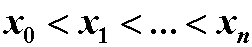的函数值为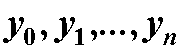，若存在一个简单函数F(x), 使，称F(x)为f(x)在区间[a,b]上的插值函数，称(xi, yi)为插值节点。若F(x)为多项式，称为多项式插值(或代数插值) ；常用的代数插值方法有：拉格朗日插值，牛顿插值。
n次代数插值：已知f(x)在n+1个点x0,x1,…,xn处的函数值为 y0,y1,…,yn ， 求一个n次多项式函数Pn(x)，使其满足： Pn(xi)=yi, (i=0,1,…,n). 若Pn(x)按下述方式构造，称为拉格朗日插值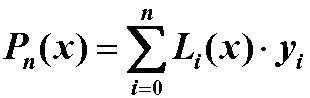其中Li(x) 为n次多项式：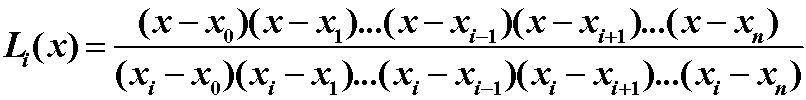称为拉格朗日插值基函数．
特别地:
（1）已知两个节点时，得线性插值多项式: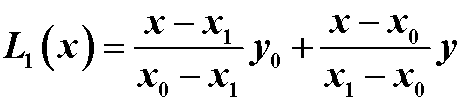（2）已知三个节点时，得抛物插值多项式: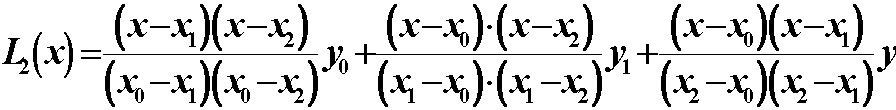（3）已知n+1个节点时，可得n次拉格朗日插值多项式。
关于代数插值：
可以看出，当节点较多时，多项式的次数增高，插值函数出现振荡，精度变低。因此，为了保证精度，在节点较多时，一般采用分段插值，但这样在分段点光滑性较差。Matlab采用的多项式插值都是分段插值法。从图形还可以看出，对解析函数，插值精度高；对有奇点的函数，插值精度低。多项式插值对靠近插值区间中点的部分插值精度高，远离中点部分精度低。
规则网点的插值
y=interp1(x0,y0,x,'method')，y是对应x插值后获得的因变量，x0和y0为初始数据的自变量和因变量
Method 的选项有 ‘nearest’, ‘next’, ‘previous’, ‘linear’,‘spline’,‘pchip’, 和 'cubic'. 缺省的机器设置为'linear'.
插值效果：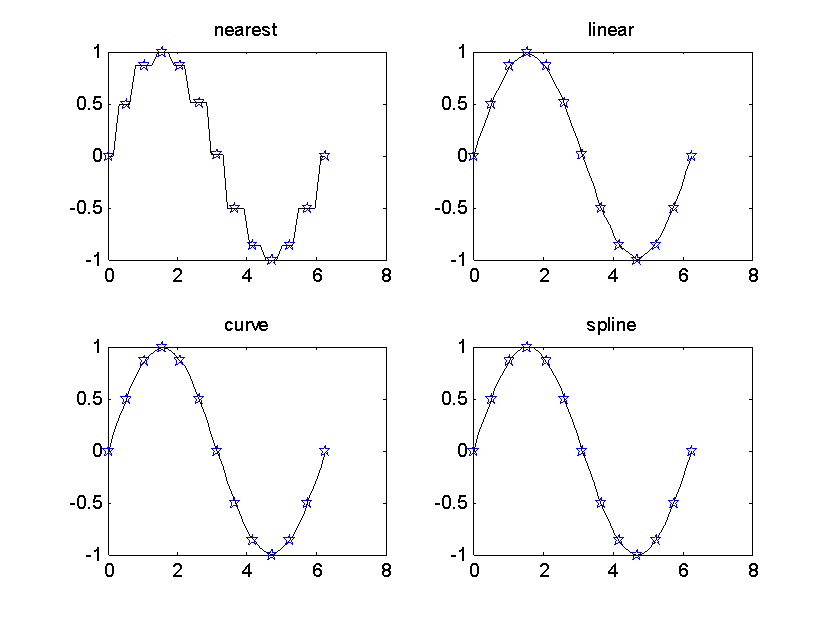参考程序：
xdata=0:pi/6:2*pi;
ydata=sin(xdata);
x=0:pi/20:2*pi;
subplot(2,2,1)
y=interp1(xdata,ydata,x,'nearest');
plot(xdata,ydata,'p',x,y,'k-')
title('nearest')
subplot(2,2,2)
y=interp1(xdata,ydata,x,'linear');
plot(xdata,ydata,'p',x,y,'k-')
title('linear')
subplot(2,2,3)
y=interp1(xdata,ydata,x,'cubic');
plot(xdata,ydata,'p',x,y,'k-')
title('cubic')
subplot(2,2,4)
y=interp1(xdata,ydata,x,'spline');
plot(xdata,ydata,'p',x,y,'k-')
title('spline')
插值误差：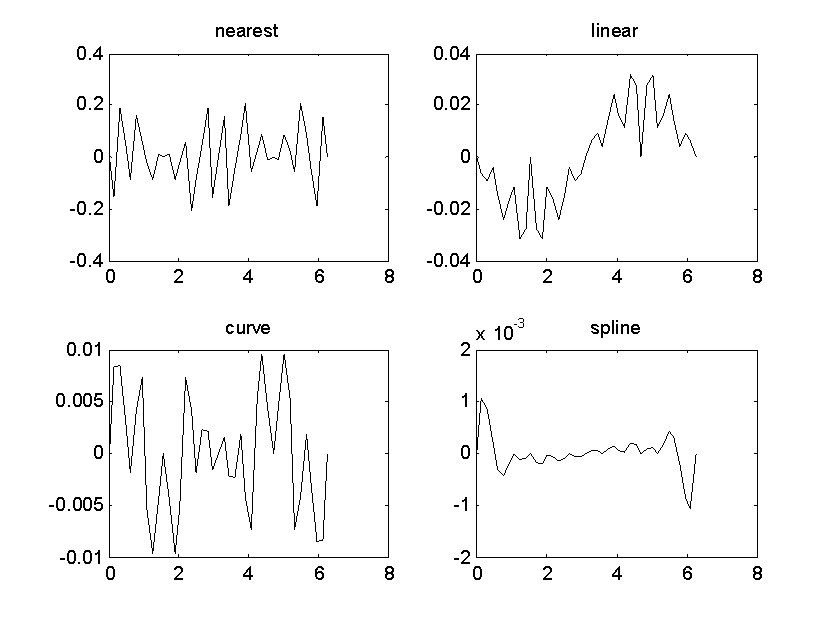参考程序：
xdata=0:pi/6:2*pi;
ydata=sin(xdata);
x=0:pi/20:2*pi;
yy=sin(x);
subplot(2,2,1)
y=interp1(xdata,ydata,x,'nearest');
plot(x,y-yy,'k-')
title('nearest')
subplot(2,2,2)
y=interp1(xdata,ydata,x,'linear');
plot(x,y-yy,'k-')
title('linear')
subplot(2,2,3)
y=interp1(xdata,ydata,x,'curve');
plot(x,y-yy,'k-')
title('curve')
subplot(2,2,4)
y=interp1(xdata,ydata,x,'spline');
plot(x,y-yy,'k-')
title('spline')
小tips：插值中使用较多的是分段线性插值和三次样条插值。
三次样条插值是解决一维插值问题最常用的方法， Matlab中实现三次样条插值的方法有：
yi=interp1(x,y,xi,’spline’)使用spline函数：
yi=spline(x, y, xi) ，效果同 1 pp=spline(x, y)，获得三次样条插值的分段多项式pp，可使用ppval计算插值使用csape函数：pp=csape(x, y)，可以添加参数选择边界条件
例1：通过实验测得某函数的一组数据如下，试作出其插值函数的图形。
解法一：
x=[0,3,5,7,9,11,12,13,14,15];
y=[0,1.2,1.65,2.1,2.15,2.0,1.85,1.65,1.55,1.25];
xi=0:0.1:15;
yi=interp1(x,y,xi, 'spline');
yi1=interp1(x,y,xi, 'linear');
yi2=interp1(x,y,xi, 'cubic');
plot(x,y,'*',xi,yi,'r-',xi,yi1,'b-',xi,yi2,'g-')
legend('节点','三次样条插值','线性插值','立方插值')
解法二：
x=[0,3,5,7,9,11,12,13,14,15];
y=[0,1.2,1.65,2.1,2.15,2.0,1.85,1.65,1.55,1.25];
xx=0: 0.1: 15;
S=csape(x, y);
%Sa=spline(x, y) ;
P=S.coefs;
%Pa=Sa.coefs;
yy=ppval(S,xx);
plot(x,y,'o',xx,yy,'r');

关于pp形式：

pp就是分段多项式，百语句形如： breaks = -5:-1; coefs = -22:-11; pp = ppmak(breaks,coefs) 其中：breaks就是各度段的端点值，-5，-4，-3，-2，-1，有问4个区间 coefs就是每段多项式的系数，答共有12个值，12/4=3，则有回4个多项式，每个多项式的最高次答数是3

二、 二元函数插值
网格节点数据插值
函数：interp2
格式：z=interp2(x0, y0, z0, x, y, ’method’)
x0,y0,z0:插值节点坐标，要求x0, y0单调；
x, y是被插值点的横坐标与纵坐标( x, y不能超过x0,y0的范围)，z是被插值点的函数值。
Method：(1)nearest 最邻近插值，(2)linear  双线性插值，(3)cubic双三次插值，默认为双线性插值。
例2：要在一山区修建公路，首先测得一些点的高程（见附件,表中数据为坐标点的高程，单位：米，y轴正向为北)绘制该地地貌图。
4800  1350  1370  1390  1400  1410  960  940  880  800  690  570  430  290  210  150  4400  1370  1390  1410  1430  1440  1140  1110  1050  950  820  690  540  380  300  210  4000  1380  1410  1430  1450  1470  1320  1280  1200  1080  940  780  620  450  370  350  3600  1420  1430  1450  1480  1500  1550  1510  1430  1300  1200  980  850  750  550  500  3200  1430  1450  1460  1500  1550  1600  1550  1600  1600  1600  1550  1500  1500  1550  1500  2800  950  1190  1370  1500  1200  1100  1550  1600  1550  1380  1070  900  1050  1150  1200  2400  910  1090  1270  1500  1200  1100  1350  1450  1200  1150  1010  880  1000  1050  1100  2000  880  1060  1230  1390  1500  1500  1400  900  1100  1060  950  870  900  930  950  1600  830  980  1180  1320  1450  1420  1400  1300  700  900  850  840  380  780  750  1200  740  880  1080  1130  1250  1280  1230  1040  900  500  700  780  750  650  550  800  650  760  880  970  1020  1050  1200  830  800  700  300  500  550  480  350  400  510  620  730  800  850  870  850  780  720  650  500  200  300  350  320  0  370  470  550  600  670  690  670  620  580  450  400  300  100  150  250  y/x  0  400  800  1200  1600  2000  2400  2800  3200  3600  4000  4400  4800  5200  5600
解：
A = xlsread('新建 XLSX 工作表.xlsx');
[xx,yy]=size(A);
Z=A([1:xx-1],[2:yy]);
x=0:400:5600;
y=4800:-400:0;
[X,Y]=meshgrid(x,y);
surf(X,Y,Z);    %离散图
figure(2);
xi=linspace(0, 5600, 80);
yi=linspace(0, 4800, 80);
[Xi,Yi]=meshgrid(xi, yi);
%%-------------------------------
Zi=interp2(X,Y,Z,Xi,Yi,'linear');    %二元插值 linear
surf(Xi,Yi,Zi);
%%-------------------------------
figure(3)
Zi=interp2(X,Y,Z,Xi,Yi,'spline');    %二元插值 spline
surf(Xi,Yi,Zi);
%%-------------------------------
figure(4)
t=0:100:1600;
[c,h]= contourf(Xi,Yi,Zi,t);    %等高线
clabel(c, h)
colormap cool
colorbar
散点数据插值函数
已知n个插值节点(xi, yi, zi), (i=1,2,…,n), 求在点(x,y)处的插值z， matlab提供函数griddata。
格式：cz=griddata(x,y,z,cx,cy,’method’)
其中x,y,z 均为n 维向量，指明所给数据点（插值节点）的横坐标、纵坐标和竖坐标。cx, cy是给定被插值点的横坐标和纵坐标，cz为相应点的竖坐标。
若cx,cy是向量，则给定以它们所确定网格点的横坐标和纵坐标，这时要求cx,cy一个为行向量一个为列向量。 编程时也可先用meshgrid将cx,cy定义成网格矩阵。
例3：在某海域测得一些点(x,y)处的水深z（英尺）如下表，船的吃水深度为5英尺，在矩形区域(75,200)×(-50,150)里那些地方船要避免进入。
x  129  140  103.5  88  185.5  195  105  157.5  107.5  77  81  162  162  117.5  y  7.5  141.5  23  147  22.5  137.5  85.5  -6.5  -81  3  56.5  -66.5  84  -33.5  z  4  8  6  8  6  8  8  9  9  8  8  9  4  9
解：
x=[129,140,103.5,88,185.5,195,105,157.5,107.5,77,81,162,162,117.5];
y=[7.5,141.5,23,147,22.5,137.5,85.5,-6.5,-81,3,56.5,-66.5,84,-33.5];
z=-[4,8,6,8,6,8,8,9,9,8,8,9,4,9];
[x1,y1]=meshgrid(75:5:200,150:-5:-50);
z1=griddata(x,y,z,x1,y1,'v4');
surf(x1,y1,z1)
figure(2)
[c,h]=contourf(x1,y1,z1);
clabel(c,h)
三、 三元函数插值
函数：interp3
格式：v = interp3(x0, y0, z0, v0 , x, y, z ,’method’)
x0,y0,z0,v0为插值数据，x,y,z为被插值的范围，v代表val
虽然三元图像不能直观的画图观察，但是可以通过切割观察剖面，配合slice函数观察切片情况
格式：slice(X,Y,Z,V,sx,sy,sz)
X,Y,Z,V为数据，sx,sy,sz可决定切片形式和位置
展开全文• ## Matlab插值方法大全

千次阅读 2018-01-11 09:10:34
功能 一维数据插值（表格查找）。该命令对数据点之间计算内插值。它找出一元函数f(x)在中间点的数值。其中函数f(x)由所给数据决定。 x：原始数据点 Y：原始数据点 xi：插值点 Yi：插值点 格式 (1)yi = interp1(x,Y,...


命令1 interp1 功能 一维数据插值（表格查找）。该命令对数据点之间计算内插值。它找出一元函数f(x)在中间点的数值。其中函数f(x)由所给数据决定。 x：原始数据点 Y：原始数据点 xi：插值点 Yi：插值点 格式 (1)yi = interp1(x,Y,xi) 返回插值向量yi，每一元素对应于参量xi，同时由向量x 与Y 的内插值决定。参量x 指定数据Y 的点。 若Y 为一矩阵，则按Y 的每列计算。yi 是阶数为length(xi)*size(Y,2)的输出矩阵。 (2)yi = interp1(Y,xi) 假定x=1:N，其中N 为向量Y 的长度，或者为矩阵Y 的行数。 (3)yi = interp1(x,Y,xi,method) 用指定的算法计算插值： ’nearest’：最近邻点插值，直接完成计算； ’linear’：线性插值（缺省方式），直接完成计算； ’spline’：三次样条函数插值。对于该方法，命令interp1 调用函数spline、ppval、mkpp、umkpp。这些命令生成一系列用于分段多项式操作的函数。命令spline 用它们执行三次样条函数插值； ’pchip’：分段三次Hermite 插值。对于该方法，命令interp1 调用函数pchip，用于对向量x 与y 执行分段三次内插值。该方法保留单调性与数据的外形； ’cubic’：与’pchip’操作相同； ’v5cubic’：在MATLAB 5.0 中的三次插值。 对于超出x 范围的xi 的分量，使用方法’nearest’、’linear’、’v5cubic’的插值算法，相应地将返回NaN。对其他的方法，interp1 将对超出的分量执行外插值算法。 (4)yi = interp1(x,Y,xi,method,’extrap’) 对于超出x 范围的xi 中的分量将执行特殊的外插值法extrap。 (5)yi = interp1(x,Y,xi,method,extrapval) 确定超出x 范围的xi 中的分量的外插值extrapval，其值通常取NaN 或0。 例1
>>x = 0:10; y = x.*sin(x); >>xx = 0:.25:10; yy = interp1(x,y,xx); >>plot(x,y,’kd’,xx,yy)
例2
>> year = 1900:10:2010; >> product = [75.995 91.972 105.711 123.203 131.669 150.697 179.323 203.212 226.505 249.633 256.344 267.893 ]; >>p1995 = interp1(year,product,1995) >>x = 1900:1:2010; >>y = interp1(year,product,x,’pchip’); >>plot(year,product,’o’,x,y)
插值结果为：
p1995 = 252.9885
命令2 interp2 功能 二维数据内插值（表格查找） 格式 (1)ZI = interp2(X,Y,Z,XI,YI) 返回矩阵ZI，其元素包含对应于参量XI 与YI（可以是向量、或同型矩阵） 的元素， 即Zi(i,j) ←[Xi(i,j),yi(i,j)]。用户可以输入行向量和列向量Xi 与Yi，此时，输出向量Zi 与矩阵meshgrid(xi,yi)是同型的。同时取决于由输入矩阵X、Y 与Z 确定的二维函数Z=f(X,Y)。参量X 与Y 必须是单调的，且相同的划分格式，就像由命令meshgrid 生成的一样。若Xi与Yi 中有在X 与Y范围之外的点，则相应地返回nan（Not a Number）。 (2)ZI = interp2(Z,XI,YI) 缺省地，X=1:n、Y=1:m，其中[m,n]=size(Z)。再按第一种情形进行计算。 (3)ZI = interp2(Z,n) 作n 次递归计算，在Z 的每两个元素之间插入它们的二维插值，这样，Z 的阶数将不断增加。interp2(Z)等价于interp2(z,1)。 (4)ZI = interp2(X,Y,Z,XI,YI,method) 用指定的算法method 计算二维插值： ’linear’：双线性插值算法（缺省算法）； ’nearest’：最临近插值； ’spline’：三次样条插值； ’cubic’：双三次插值。 例3：
>>[X,Y] = meshgrid(-3:.25:3); >>Z = peaks(X,Y); >>[XI,YI] = meshgrid(-3:.125:3); >>ZZ = interp2(X,Y,Z,XI,YI); >>surfl(X,Y,Z);hold on; >>surfl(XI,YI,ZZ+15) >>axis([-3 3 -3 3 -5 20]);shading flat >>hold off
例4：
>>years = 1950:10:1990; >>service = 10:10:30; >>wage = [150.697 199.592 187.625 179.323 195.072 250.287 203.212 179.092 322.767 226.505 153.706 426.730 249.633 120.281 598.243]; >>w = interp2(service,years,wage,15,1975)
插值结果为：
w = 190.6288
命令3 interp3 功能 三维数据插值（查表） 格式 (1)VI = interp3(X,Y,Z,V,XI,YI,ZI) 找出由参量X,Y,Z决定的三元函数V=V(X,Y,Z)在点（XI,YI,ZI）的值。参量XI,YI,ZI 是同型阵列或向量。若向量参量XI,YI,ZI 是不同长度，不同方向（行或列）的向量，这时输出参量VI 与Y1,Y2,Y3 为同型矩阵。其中Y1,Y2,Y3 为用命令meshgrid(XI,YI,ZI)生成的同型阵列。若插值点(XI,YI,ZI)中有位于点(X,Y,Z)之外的点，则相应地返回特殊变量值NaN。 (2)VI = interp3(V,XI,YI,ZI) 缺省地， X=1:N ，Y=1:M， Z=1：P ，其中，[M,N,P]=size(V)，再按上面的情形计算。 (3)VI = interp3(V,n) 作n 次递归计算，在V 的每两个元素之间插入它们的三维插值。这样，V 的阶数将不断增加。interp3(V)等价于interp3(V,1)。 (4)VI = interp3(……,method) %用指定的算法method 作插值计算： ‘linear’：线性插值（缺省算法）； ‘cubic’：三次插值； ‘spline’：三次样条插值； ‘nearest’：最邻近插值。 说明 在所有的算法中，都要求X,Y,Z 是单调且有相同的格点形式。当X,Y,Z 是等距且单调时，用算法’*linear’，’*cubic’，’*nearest’，可得到快速插值。 例5
>>[x,y,z,v] = flow(20); >>[xx,yy,zz] = meshgrid(.1:.25:10, -3:.25:3, -3:.25:3); >>vv = interp3(x,y,z,v,xx,yy,zz); >>slice(xx,yy,zz,vv,[6 9.5],[1 2],[-2 .2]); shading interp;colormap cool
命令4 interpft 功能 用快速Fourier 算法作一维插值 格式 (1)y = interpft(x,n) 返回包含周期函数x 在重采样的n 个等距的点的插值y。若length(x)=m，且x 有采样间隔dx，则新的y 的采样间隔dy=dx*m/n。注意的是必须n≥m。若x 为一矩阵，则按x 的列进行计算。返回的矩阵y 有与x 相同的列数，但有n 行。 (2)y = interpft(x,n,dim) 沿着指定的方向dim 进行计算 命令5 griddata 功能 数据格点 格式 (1)ZI = griddata(x,y,z,XI,YI) 用二元函数z=f(x,y)的曲面拟合有不规则的数据向量x,y,z。griddata 将返回曲面z 在点（XI,YI）处的插值。曲面总是经过这些数据点（x,y,z）的。输入参量（XI,YI）通常是规则的格点（像用命令meshgrid 生成的一样）。XI 可以是一行向量，这时XI 指定一有常数列向量的矩阵。类似地，YI 可以是一列向量，它指定一有常数行向量的矩阵。 (2)[XI,YI,ZI] = griddata(x,y,z,xi,yi) 返回的矩阵ZI 含义同上，同时，返回的矩阵XI,YI 是由行向量xi 与列向量yi 用命令meshgrid 生成的。 (3)[XI,YI,ZI] = griddata(…….,method) 用指定的算法method 计算： ‘linear’：基于三角形的线性插值（缺省算法）； ‘cubic’： 基于三角形的三次插值； ‘nearest’：最邻近插值法； ‘v4’：MATLAB 4 中的griddata 算法。 命令6 spline 功能 三次样条数据插值 格式 (1)yy = spline(x,y,xx) 对于给定的离散的测量数据x,y（称为断点），要寻找一个三项多项式y = p(x) ，以逼近每对数据(x,y)点间的曲线。过两点(xi, yi) 和(xi+1, yi+1) 只能确定一条直线，而通过一点的三次多项式曲线有无穷多条。为使通过中间断点的三次多项式曲线具有唯一性，要增加两个条件（因为三次多项式有4 个系数）： a．三次多项式在点(xi, yi) 处有： p&cent;i(xi) = p&cent;i(xi) ； b．三次多项式在点(xi+1, yi+1) 处有： p&cent;i(xi+1) = pi&cent;(xi+1) ； c．p(x)在点(xi, yi) 处的斜率是连续的（为了使三次多项式具有良好的解析性，加上的条件）； d．p(x)在点(xi, yi) 处的曲率是连续的； 对于第一个和最后一个多项式，人为地规定如下条件： ①． p&cent;1&cent;(x) = p&cent;2&cent;(x) ②． p&cent;n&cent;(x) = p&cent;n&cent;-1(x) 上述两个条件称为非结点(not-a-knot)条件。
该命令用三次样条插值计算出由向量x 与y 确定的一元函数y=f(x)在点xx 处的值。若参量y 是一矩阵，则以y 的每一列和x 配对，再分别计算由它们确定的函数在点xx 处的值。则yy 是一阶数为length(xx)*size(y,2)的矩阵。 (2)pp = spline(x,y) 返回由向量x 与y 确定的分段样条多项式的系数矩阵pp，它可用于命令ppval、unmkpp 的计算。 例6 对离散地分布在y=exp(x)sin(x)函数曲线上的数据点进行样条插值计算：
>>x = [0 2 4 5 8 12 12.8 17.2 19.9 20]; y = exp(x).*sin(x); >>xx = 0:.25:20; >>yy = spline(x,y,xx); >>plot(x,y,’o’,xx,yy)
命令7 interpn 功能 n 维数据插值（查表） 格式 (1)VI = interpn(X1,X2,,,Xn,V,Y1,Y2,⋯,Yn) %返回由参量X1,X2,…,Xn,V 确定的n 元函数V=V(X1,X2,…,Xn)在点（Y1,Y2,…,Yn）处的插值。参量Y1,Y2,…,Yn 是同型的矩阵或向量。若Y1,Y2,…,Yn 是向量，则可以 是不同长度，不同方向（行或列）的向量。它们将通过命令ndgrid生成同型的矩阵， 再作计算。若点(Y1,Y2,…,Yn) 中有位于点（X1,X2,…,Xn）之外的点，则相应地返回特殊变量NaN。 VI = interpn(V,Y1,Y2,⋯,Yn) %缺省地，X1=1:size(V,1)，X2=1:size(V,2)，… ， Xn=1:size(V,n)，再按上面的情形计算。 VI = interpn(V,ntimes) %作ntimes 次递归计算，在V 的每两个元素之间插入它们的n 维插值。这样，V 的阶数将不断增加。interpn(V) 等价于interpn(V, 1)。 VI = interpn(⋯,method) %用指定的算法method 计算： ‘linear’：线性插值（缺省算法）； ‘cubic’：三次插值； ‘spline’：三次样条插值法； ‘nearest’：最邻近插值算法。 命令8 meshgrid 功能 生成用于画三维图形的矩阵数据。 格式 [X,Y] = meshgrid(x,y) 将由向量x，y（可以是不同方向的）指定的区域[min(x)，max(x) ， min(y) ， max(y)] 用直线x=x(i),y=y(j) （ i=1,2,…,length(x) ，j=1,2,…,length(y)）进行划分。这样，得到了length(x)*length(y)个点， 这些点的横坐标用矩阵X 表示，X 的每个行向量与向量x 相同；这些点的纵坐标用矩阵Y 表示，Y 的每个列向量与向量y 相同。其中X,Y可用于计算二元函数z=f(x,y)与三维图形中xy 平面矩形定义域的划分或 曲面作图。 [X,Y] = meshgrid(x) %等价于[X,Y]=meshgrid(x,x)。 [X,Y,Z] = meshgrid(x,y,z) %生成三维阵列X,Y,Z，用于计算三元函数v=f(x,y,z)或三维容积图。 例7
[X,Y] = meshgrid(1:3,10:14)
计算结果为：
X = 1 2 3 1 2 3 1 2 3 1 2 3 1 2 3 Y = 10 10 10 11 11 11 12 12 12 13 13 13 14 14 14
命令9 ndgrid
功能 生成用于多维函数计算或多维插值用的阵列 格式 [X1,X2,…,Xn] = ndgrid(x1,x2,…,xn) %把通过向量x1,x2,x3…,xn 指定的区域转换为数组x1,x2,x3,…,xn 。这样， 得到了 length(x1)*length(x2)*…*length(xn)个点，这些点的第一维坐标用矩阵X1 表 示，X1 的每个第一维向量与向量x1 相同；这些点的第二维坐标用矩阵X2 表示，X2 的每个第二维向量与向量x2 相同；如此等等。 其中X1,X2,…,Xn 可用于计算多元函数y=f(x1,x2,…,xn)以及多维插值命令用到的阵列。 [X1,X2,…,Xn] = ndgrid(x) %等价于[X1,X2,…,Xn] = ndgrid(x,x,…,x) 命令10 table1 功能 一维查表 格式 Y = table1(TAB,X0) %返回用表格矩阵TAB 中的行线性插值元素，对X0（TAB的第一列查找X0）进行线性插值得到的结果Y。矩阵TAB 是第一列包含 关键值，而其他列包含数据的矩阵。X0 中的每一元素将相应地返回一线性插值行向量。矩阵TAB 的第一列必须是单调的。 例8
>>tab = [(1:4)’ hilb(4)] >>y = table1(tab,[1 2.3 3.6 4])
查表结果为：
>>tab = [(1:4)’ hilb(4)] >>y = table1(tab,[1 2.3 3.6 4])
这里写链接内容
展开全文• 用于matlab的反距离权重插值函数，是比较方便的插值方法之一 用于matlab的反距离权重插值函数，是比较方便的插值方法之一
• 一.1维插值函数 1.通用接口: 进行1维插值:vq=interp1([x,]v,xq[,method,extrapolation) #参数说明: x,v:分别指定样本点的x,y值;x默认为[1,2...length(v)](v为向量)或[1,2...size(v,1)](v为高维数组) #注意:...
一.插值 1.1维插值函数 (1)通用接口:
进行1维插值:vq=interp1([x,]v,xq[,method,extrapolation)
#参数说明:
x,v:分别指定样本点的x,y值;x默认为[1,2...length(v)](v为向量)或[1,2...size(v,1)](v为高维数组)
#注意:要求x是单调的
xq:指定查询点(即求该点的插值)
method:指定插值方法;可为'linear'(默认值;分段线性插值)/'nearest'(最近邻点插值)/'next'(下1个邻点插值)/'previous'(上1个邻点插值)/'pchip'(保形分段3次插值)/'cubic'(保形分段3次插值)/'v5cubic'(用于MATLAB5的3次卷积)/'makima'(修正了Akima公式的3次埃尔米特插值)/'spline'(3次样条插值)
#注意:x等距时可使用快速插值,此时指定method为'*linear'等
extrapolation:指定外插策略;可为'extrap'(使用method计算)/标量值(返回该值)/NaN(默认值;返回NaN)
#即当xq落在x外,如何处理
vq:返回插值

#实例:
>> x=[1,2,3,4];
>> v=[1,-1,3,9];
>> interp1(x,v,3.5)

ans =

6

>> v=[1,1,1;-1,-1,-1;3,3,3;9,9,9];
>> interp1(v,3.5)

ans =

6     6     6


(2)3次样条插值:
进行3次样条插值:s=spline(x,y[,xq])
#参数说明:
x,y:分别指定样本点的x,y值

######################################################################################################################

csape()

(3)分段3次埃尔米特插值(保型分段3次插值):
pchip()

(4)分段多项式插值:
mkpp()

2.2维插值 (1)通用接口:
interp2()

二.拟合 1.1元多项式曲线拟合(Polynomial Curve Fitting):
进行1元多项式曲线拟合:[p,S,mu] = polyfit(x,y,n)
#参数说明:
x,y:分别指定数据的x,y值
n:指定多项式的次数
p:返回按降幂排列的多项式系数
S:返回1个结构体,可用作polyval()的输入来获取误差估计值
mu:返回1个二元素向量,mu(1)为mean(x),mu(2)为std(x)
#要求返回mu时,polyfit()会对x进行Z-Score Standarlization,这会改善多项式和拟合算法的数值属性

#实例:
>> polyfit([1,2,3],[1,4,9],1)

ans =

4.0000   -3.3333

>> [p,S,mu]=polyfit([1,2,3],[1,4,9],1)

p =

4.0000    4.6667

S =

包含以下字段的 struct:

R: [2×2 double]
df: 1
normr: 0.8165

mu =

2
1


2.多元线性回归:
进行多元线性回归:[b,bint,r,rint,stats] = regress(y,X[,alpha])
#设多元线性方程为y=b1*x1+b2*x2+...+bn*xn
#参数说明:
y:指定数据点的y坐标;为column array
X:指定数据点的x坐标;为matrix(每列对应1个变量)
#y,X的行数应相等;X(:,1)对应x1,X(:,2)对应x2...;若方程有常数项,应有某列全为1
alpha:指定置信水平;默认为0.05
#将使用100*(1-alpha)%置信水平
b:返回超平面的各个系数构成的向量
#b(1)为b1,b(2)为b2...
bint:返回1个矩阵,其中包含系数估计值的95%置信区间
r:返回由残差组成的向量
rint:返回1个矩阵,其中包含可用于诊断离群值的区间
stats:返回1个向量,其中包含R2统计量/F统计量及其p值,以及误差方差的估计值
#X应包含1个全为1组成的列,以便正确计算模型统计量

3.曲线拟合工具箱(Curve Fitting Toolbox):
>> cftool%见下图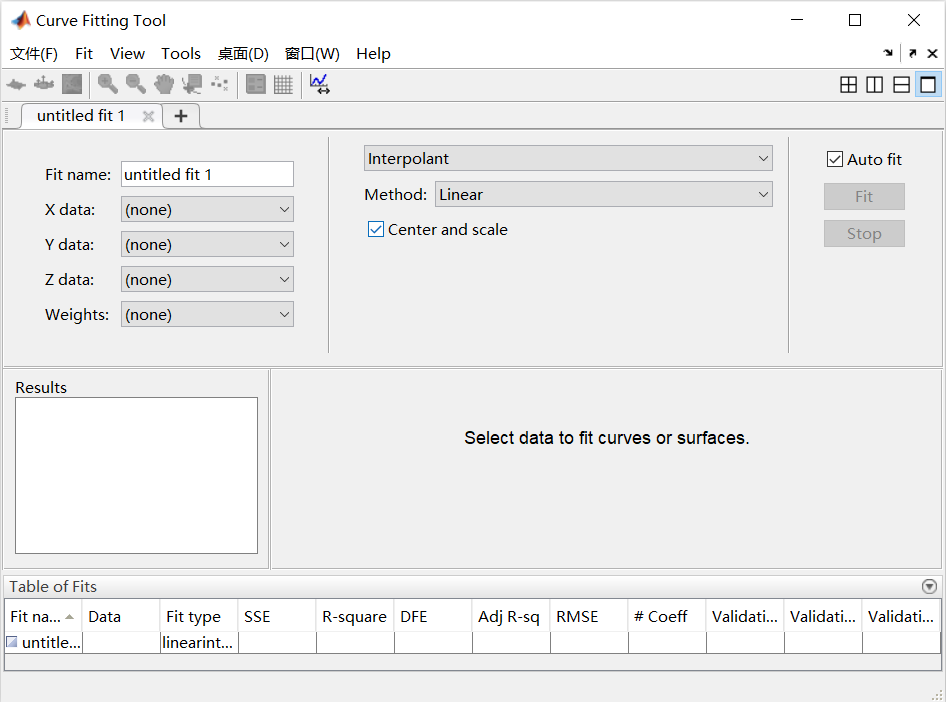展开全文• 分别通过待定系数插值、拉格朗日插值、三次和五次数据回归的方法对凸轮台工程案例进行工程数值计算。计算原理，流程图，程序代码，结果。
• 根据沈祖仪的《水轮机调节》》一书及其他相关文献建立的水轮机传递系数建模与插值模型
• ## MATLAB之多项式插值

千次阅读 2020-04-12 09:28:31
MATLAB之多项式插值 一、算法原理 函数解析式未知，但已知一些列点的函数值。如下表所示，对于n+1个点，我们可以找到一个次数不超过n的插值多项式。 ，称f为x的n次插值多项式。 x0 x1 x2 x3 ...... ...算法
• 一、interp1 1、函数简介 MATLAB中的插值函数为interp1，其调用格式为：yi= interp1(x,y,xi,'... 'method'表示采用的插值方法，MATLAB提供的插值方法有几种： 'nearest'是最邻近插值， 'linear'线性插值； 'sp...算法
• matlab多项式插值方法实现 最近学习计算方法，需要实现一些方法。但是我又不愿意去直接调用matlab原有的方法，因此在这里实现了一些插值方法。 拉格朗日插值法 function yout = LagZ(xmatrix, ymatrix, x) yout=0; n...计算方法 算法
• 插值法 插值法又称“内插法”，是利用函数f (x)在某区间中已知的若干点的函数值，作出适当的特定函数，在区间的其他点上用这特定函数的值作为函数f (x)的近似值，这种方法称为插值法。如果这特定函数是多项式，就称...
• ## Matlab插值和拟合

千次阅读 2015-06-10 16:05:09
数值插值 一维插值 YI=interp1(X,Y,XI,METHOD) X、Y为采样点和样本值，XI为表示要插值的点，METHOD默认是线性插值'linear' METHOD可以取 'nearest' - 邻近点插值法 'linear' - 线性插值 'spline' - 三次样条插值...
• ## MATLAB插值函数interp1

万次阅读 多人点赞 2017-04-15 00:51:02
插值法  插值法又称“内插法”，是利用函数f (x)在某区间中已知的若干点的函数值，作出适当的特定函数，在区间的其他点上用这特定函数的值作为函数f (x)的近似值，这种方法称为插值法。如果这特定函数是多项式，...
• 克里金插值matlab程序 克里金(Kriging)插值法又称空间自协方差最佳插值法，它是以南非矿业工程师D．G．Krige的名字命名的一种最优内插法。克里金法广泛地应用于地下水模拟、土壤制图等领域，是一种很有用的地质统计...
• Matlab系列之插值与拟合前言介绍插值一维插值二维插值拟合实例应用更多精彩，等你发现~ 前言 在前几天看了一个科幻的影视剧，名字叫“开发者”，有8集，时间也不短，还没来得及看完，但是在开始的几集里头，就出现了...数据分析
• 此代码提供具有第一条线性样条的二次样条插值。... 此代码的输出是向量（Coeff）中的二次方程系数，该系数按[a1; b1; c1; a2; b2; c2 .... ,, an; bn; cn]排序。 生成的图包含红色圆圈中的插值数据，二次样条。
• MATLAB平台中多项式插值系数被传送到SU2代码库的两个独立部分，即作为网格运动求解器和用于计算气动载荷以确保广义位移的求解器和分别计算广义的空气动力。 下图概述了五阶多项式插值映射在NASTRAN中设计的结构...
• ## Matlab中插值函数汇总及使用说明

万次阅读 多人点赞 2018-09-12 19:44:52
MATLAB中的插值函数为interp1，其调用格式为： yi= interp1(x,y,xi,'method')  其中x，y为插值点，yi为在被插值点xi处的插值结果；x,y为向量， 'method'表示采用的插值方法，MATLAB提供的插...
• MATLAB：多项式处理 1.多项式计算 2. 数据插值数学建模
• 可以通过给定的正因子缩放给定的图像。... 如果图像文件的名称是 image.jpg 并且需要的缩放系数是 2.23， 在命令窗口上运行以下命令。 Nearest_neighbor_zoom('image.jpg',2.23); 留下你的评论...
• matlab代码 [x,y]=meshgrid(-3:1:3); z=peaks(x,y) [xi,yi]=meshgrid(-3:0.25:3); figure(1) surfc(x,y,z) title('原始数据') zi1=interp2(x,y,z,xi,yi,'spline') zi2=interp2(x,y,z,xi,yi,'linear') zi3=interp2(x,...数据分析
• matlab编写的三次样条插值源代码，最终画出拟合图像，并输出拟合函数的系数三次样条差值
• ## matlab克里格插值

千次阅读 多人点赞 2020-04-18 22:45:59
为履行前期承若，现在公开matlab的克里格插值的代码。 原理介绍 普通克里格法：假定Z(x)Z(x)Z(x)是满足本征假设的一个随机过程，该随机过程有nnn个观测值z(xi)z(xi)z(xi)，要预测未采样点x0处的值，则线性预测值Z∗...线性代数 矩阵 算法 机器学习 数据挖掘
• 1、拉格朗日插值 新建如下函数： function y=lagrange(x0,y0,x) %拉格朗日插值函数 ...%m 个插值点以数组 x 输入,输出数组 y 为 m 个插值 n=length(x0);m=length(x); for i=1:m z=x(i); s
• ## matlab插值与拟合

千次阅读 2018-10-11 21:52:18
分段线性插值 y=interp1(x0,y0,x,'method') method的值可以为'nearest','linear','spline','cubic',预设方法是linear。如果数据的变化较大，以 spline函数内插所形成的曲线最平滑 ，所以效果最好。而三次方程式所...
• 该函数使用拉格朗日方法找到通过所有这些点的 N-1 阶多项式，并在 P 中返回定义该多项式的 N 个系数。 然后，polyval(P,X) = Y。 R 返回结果多项式的 N-1 个极值/拐点的 x 坐标（其导数的根），S 返回多项式在这些...
• ## Matlab中插值函数汇总和使用说明

万次阅读 多人点赞 2016-08-25 09:50:28
...MATLAB中的插值函数为interp1，其调用格式为： yi= interp1(x,y,xi,'method')  其中x，y为插值点，yi为在被插值点xi处的插值结果；x,y为向量， 'method'表示采用的插值方法，MATLAB...

# matlab插值系数matlab 订阅